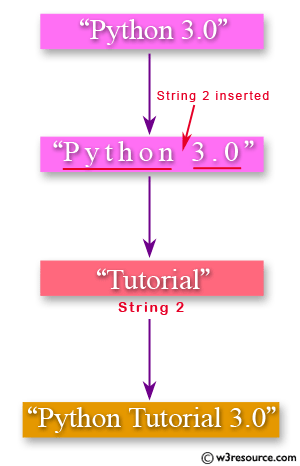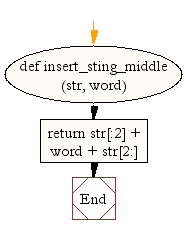﻿ Python: Insert a string in the middle of a string - w3resource

# Python: Insert a string in the middle of a string

## Python String: Exercise-16 with Solution

Write a Python function to insert a string in the middle of a string.Sample Solution:-

Python Code:

``````def insert_sting_middle(str, word):
return str[:2] + word + str[2:]

print(insert_sting_middle('[[]]', 'Python'))
print(insert_sting_middle('{{}}', 'PHP'))
print(insert_sting_middle('<<>>', 'HTML'))
```
```

Sample Output:

```[[Python]]
{{PHP}}
<<HTML>>
```

Flowchart:## Visualize Python code execution:

The following tool visualize what the computer is doing step-by-step as it executes the said program:

Python Code Editor:

Have another way to solve this solution? Contribute your code (and comments) through Disqus.

What is the difficulty level of this exercise?

Test your Programming skills with w3resource's quiz.

﻿

## Python: Tips of the Day

Decapitalizes the first letter of a string:

Example:

```def tips_decapitalize(s, upper_rest=False):
return s[:1].lower() + (s[1:].upper() if upper_rest else s[1:])
print(tips_decapitalize('PythonTips'))
print(tips_decapitalize('PythonTips', True))
```

Output:

```pythonTips
pYTHONTIPS
```

We are closing our Disqus commenting system for some maintenanace issues. You may write to us at reach[at]yahoo[dot]com or visit us at Facebook